Check Full Chapter Explained - Continuity and Differentiability - Application of Derivatives (AOD) Class 12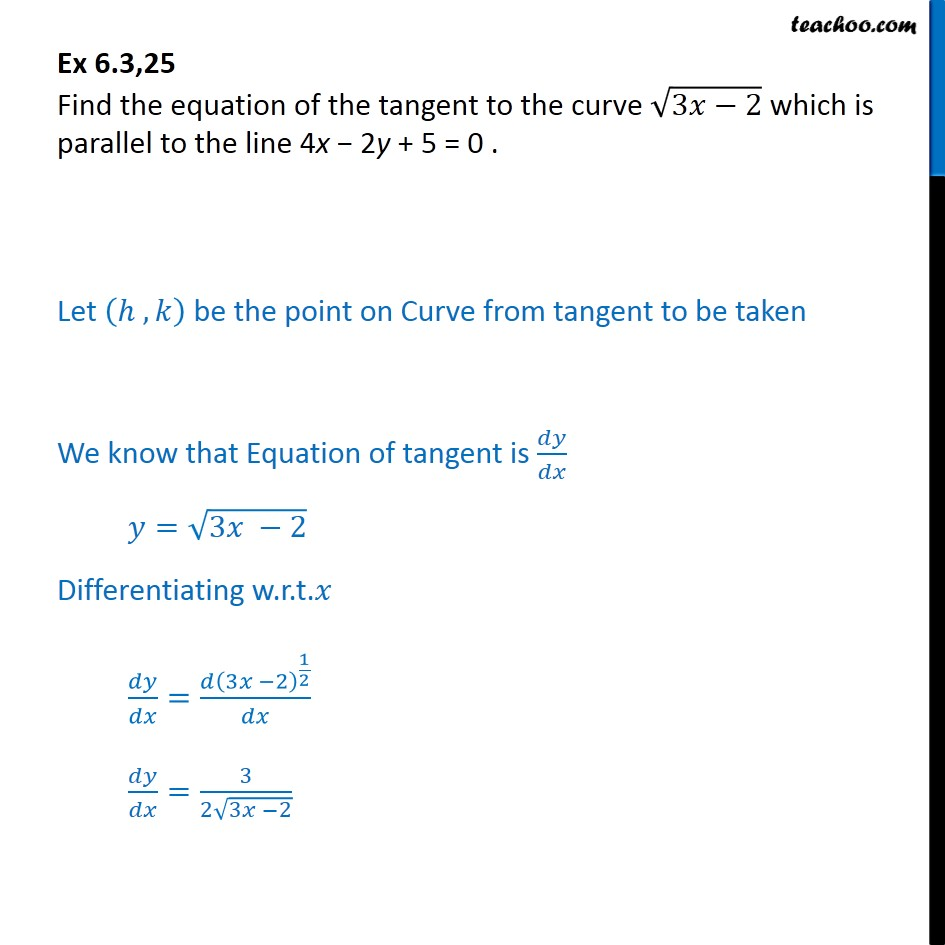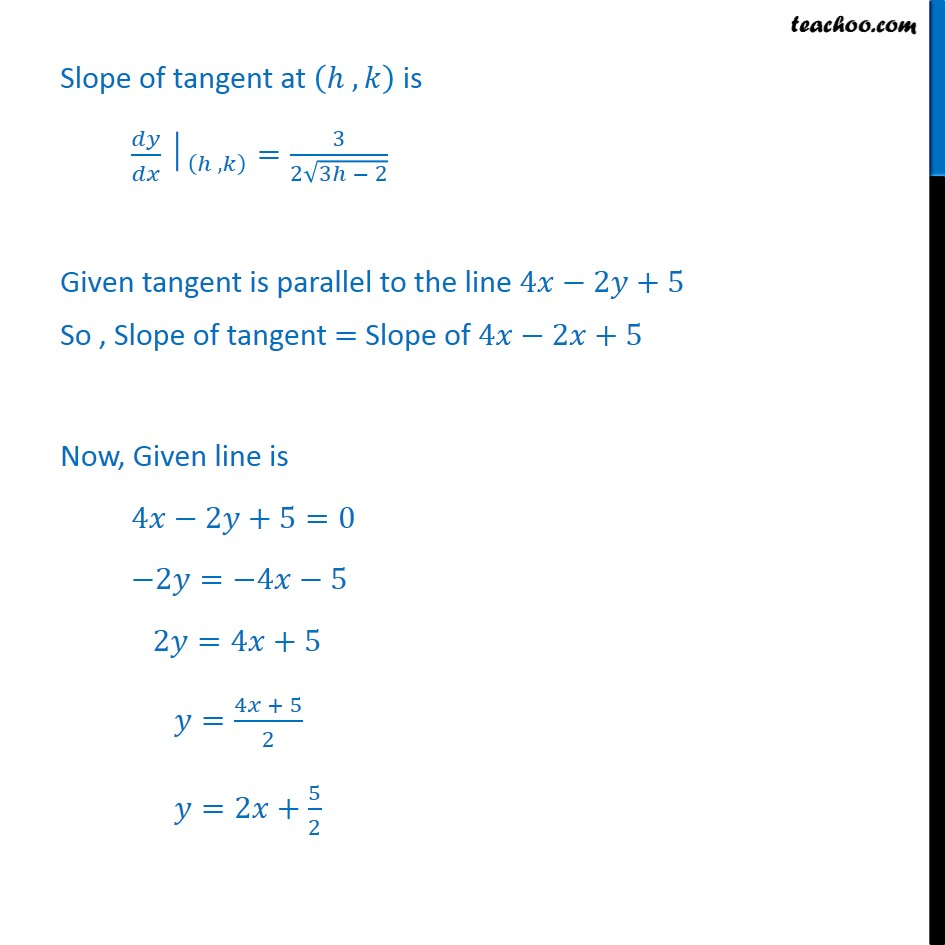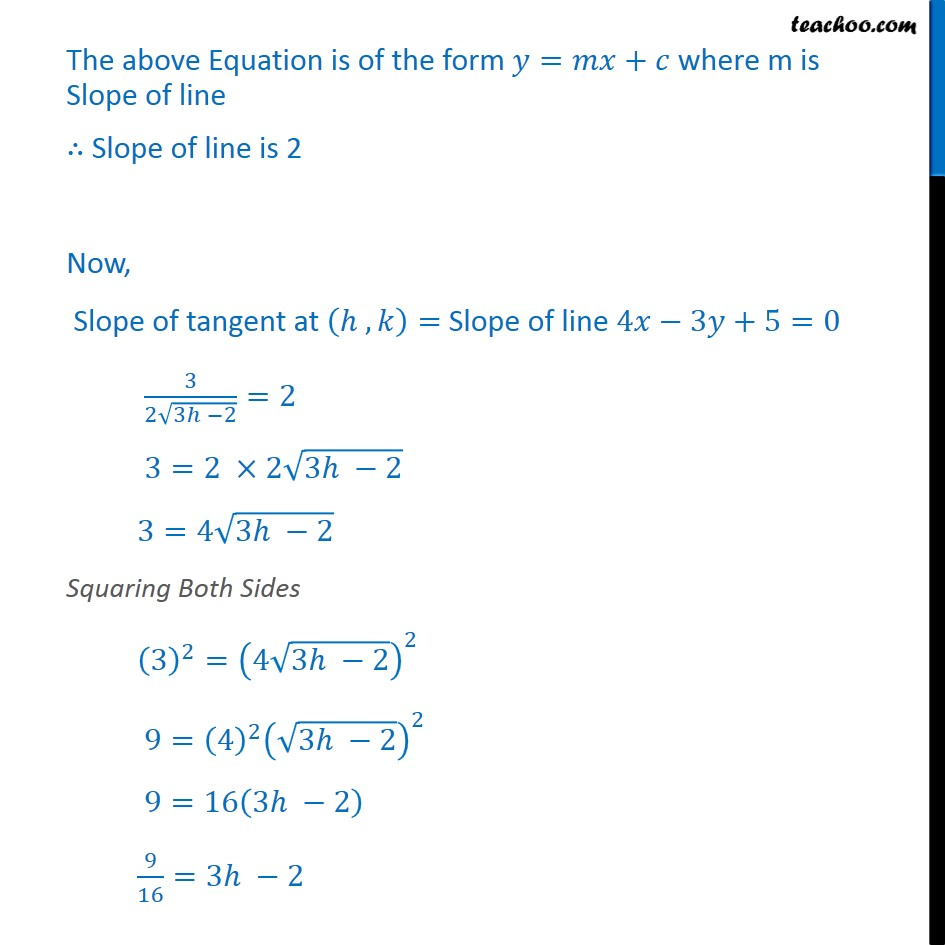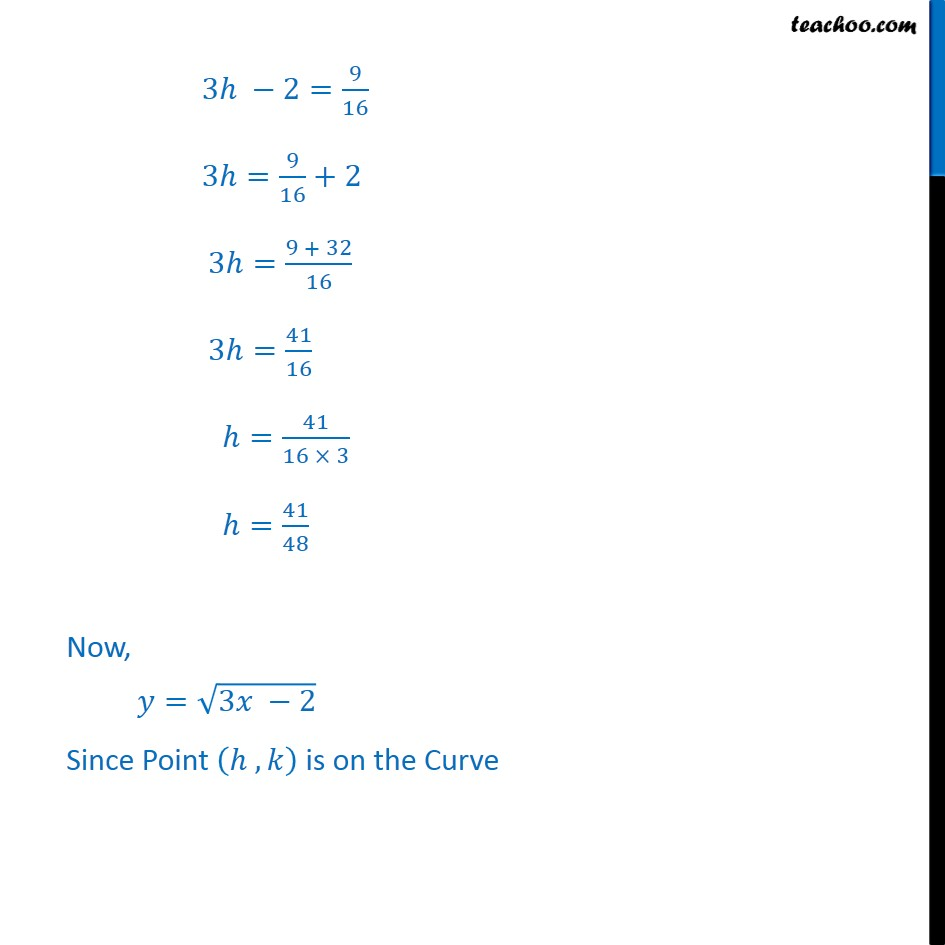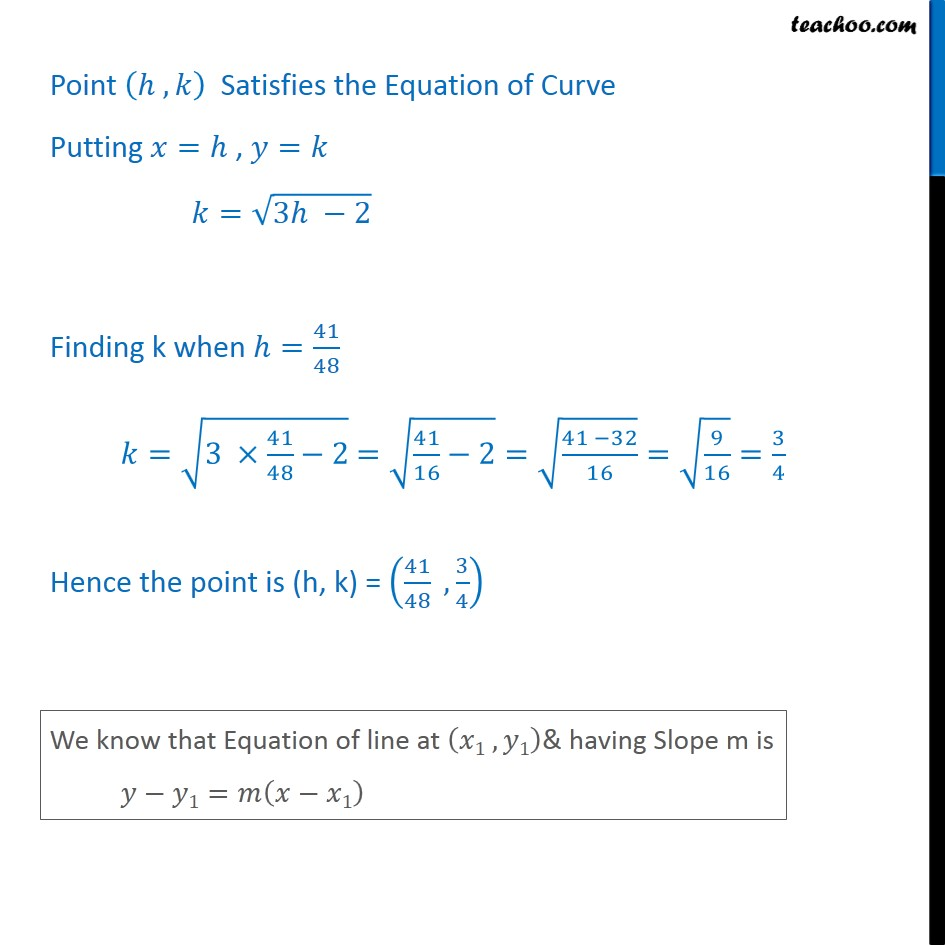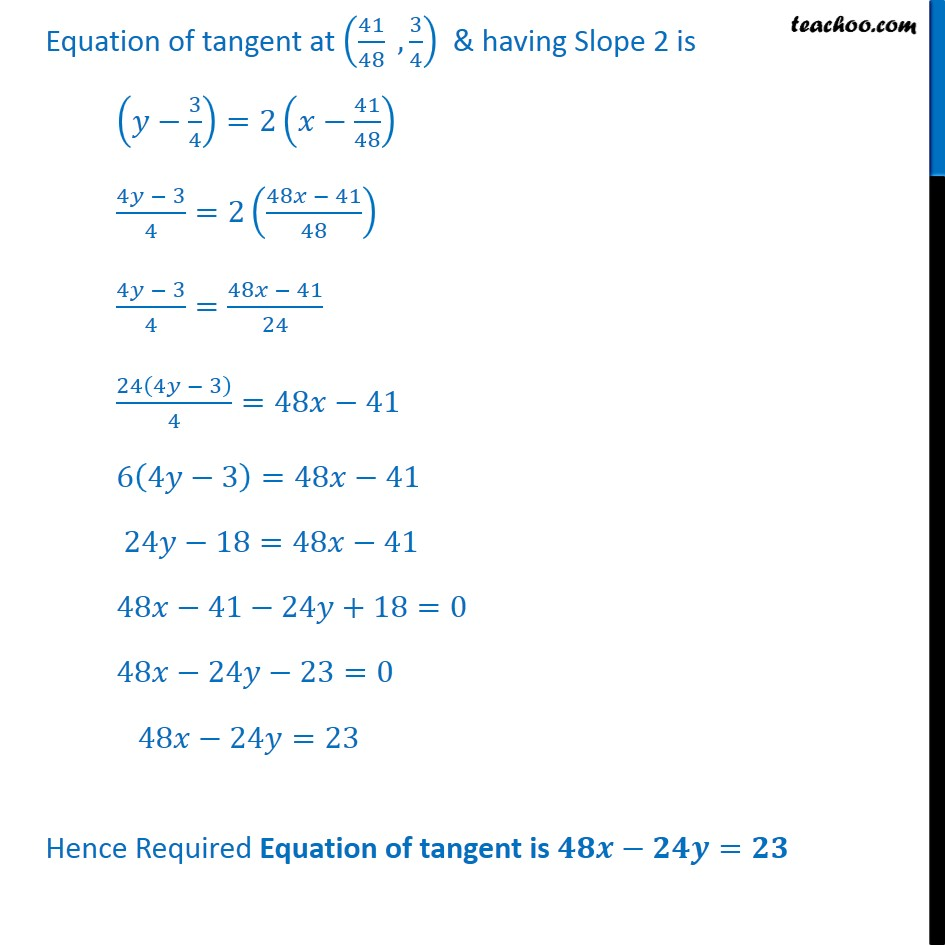1. Chapter 6 Class 12 Application of Derivatives
2. Serial order wise
3. Ex 6.3

Transcript

Ex 6.3,25 Find the equation of the tangent to the curve ﷮3𝑥−2﷯ which is parallel to the line 4x − 2y + 5 = 0 . Let ℎ , 𝑘﷯ be the point on Curve from tangent to be taken We know that Equation of tangent is 𝑑𝑦﷮𝑑𝑥﷯ 𝑦= ﷮3𝑥 −2﷯ Differentiating w.r.t.𝑥 𝑑𝑦﷮𝑑𝑥﷯= 𝑑 3𝑥 −2﷯﷮ 1﷮2﷯﷯﷮𝑑𝑥﷯ 𝑑𝑦﷮𝑑𝑥﷯= 3﷮2 ﷮3𝑥 −2﷯﷯ Slope of tangent at ℎ , 𝑘﷯ is 𝑑𝑦﷮𝑑𝑥﷯│﷮ ℎ , 𝑘﷯﷯= 3﷮2 ﷮3ℎ − 2﷯﷯ Given tangent is parallel to the line 4𝑥−2𝑦+5 So , Slope of tangent = Slope of 4𝑥−2𝑥+5 Now, Given line is 4𝑥−2𝑦+5=0 −2𝑦=−4𝑥−5 2𝑦=4𝑥+5 𝑦= 4𝑥 + 5﷮2﷯ 𝑦=2𝑥+ 5﷮2﷯ The above Equation is of the form 𝑦=𝑚𝑥+𝑐 where m is Slope of line ∴ Slope of line is 2 Now, Slope of tangent at ℎ , 𝑘﷯= Slope of line 4𝑥−3𝑦+5=0 3﷮2 ﷮3ℎ −2﷯﷯=2 3=2 ×2 ﷮3ℎ −2﷯ 3=4 ﷮3ℎ −2﷯ Squaring Both Sides 3﷯﷮2﷯= 4 ﷮3ℎ −2﷯﷯﷮2﷯ 9= 4﷯﷮2﷯ ﷮3ℎ −2﷯﷯﷮2﷯ 9=16 3ℎ −2﷯ 9﷮16﷯=3ℎ −2 3ℎ −2= 9﷮16﷯ 3ℎ= 9﷮16﷯+2 3ℎ= 9 + 32﷮16﷯ 3ℎ= 41﷮16﷯ ℎ= 41﷮16 × 3﷯ ℎ= 41﷮48﷯ Now, 𝑦= ﷮3𝑥 −2﷯ Since Point ℎ , 𝑘﷯ is on the Curve Point ℎ , 𝑘﷯ Satisfies the Equation of Curve Putting 𝑥=ℎ , 𝑦=𝑘 𝑘= ﷮3ℎ −2﷯ Finding k when ℎ= 41﷮48﷯ 𝑘= ﷮3 × 41﷮48﷯−2﷯ = ﷮ 41﷮16﷯−2﷯= ﷮ 41 −32﷮16﷯﷯= ﷮ 9﷮16﷯﷯= 3﷮4﷯ Hence the point is (h, k) = 41﷮48﷯ , 3﷮4﷯﷯ Equation of tangent at 41﷮48﷯ , 3﷮4﷯﷯ & having Slope 2 is 𝑦− 3﷮4﷯﷯=2 𝑥− 41﷮48﷯﷯ 4𝑦 − 3﷮4﷯=2 48𝑥 − 41﷮48﷯﷯ 4𝑦 − 3﷮4﷯= 48𝑥 − 41﷮24﷯ 24 4𝑦 − 3﷯﷮4﷯=48𝑥−41 6 4𝑦−3﷯=48𝑥−41 24𝑦−18=48𝑥−41 48𝑥−41−24𝑦+18=0 48𝑥−24𝑦−23=0 48𝑥−24𝑦=23 Hence Required Equation of tangent is 𝟒𝟖𝒙−𝟐𝟒𝒚=𝟐𝟑

Ex 6.3